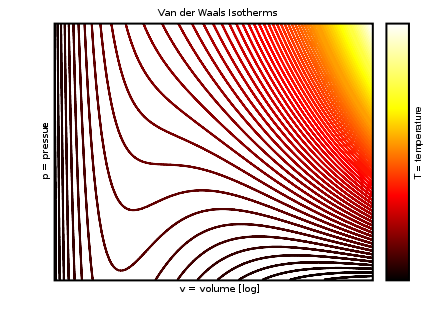## Van der Waals Equation

#### Learning Objective

• Distinguish the van der Waals equation from the Ideal Gas Law.

#### Key Points

• The van der Waals equation is an equation of state that corrects for two properties of real gases: the excluded volume of gas particles and attractive forces between gas molecules.
• The van der Waals equation is frequently presented as: $(P + \frac{an^2}{V^2})(V-nb) = nRT$ .
• The constants a and b represent the magnitude of intermolecular attraction and excluded volume respectively, and are specific to a particular gas.

#### Terms

• van der waals equationa relation between particles of a fluid that have a non-zero volume and a pairwise attractive inter-particle force
• ideal gas lawan equation describing the properties of an ideal gas, generally presented as PV = nRT

The Ideal Gas Law is based on the assumptions that
gases are composed of point masses that undergo perfectly elastic
collisions.  However, real gases deviate
from those assumptions at low temperatures or high pressures.  Imagine a container where the pressure is
increased.  As the pressure increases,
the volume of the container decreases.  The
volume occupied by the gas particles is no longer negligible compared to the
volume of the container and the volume of the gas particles needs to be taken
into account.  At low temperatures, the
gas particles have lower kinetic energy and do not move as fast.  The gas particles are affected by the
intermolecular forces acting on them, which leads to inelastic collisions between
them.  This leads to fewer collisions
with the container and a lower pressure than what is expected from an ideal
gas.

## The van der Waals Equation and P-V Isotherms

Derived by Johannes Diderik van der Waals in 1873, the van der Waals equation modifies the Ideal Gas Law; it predicts the properties of real gases by describing particles of non-zero volume governed by pairwise attractive forces. This equation of state is presented as:

$(P + \frac{an^2}{V^2})(V-nb) = nRT$

where P is the pressure, V is the volume, R is the universal gas constant, and T is the absolute temperature. Isotherm (plots of pressure versus volume at constant temperature) can be produced using the van der Waals model.van der Waals isothermsThis graph demonstrates the relationship between pressure, volume, and temperature based on the van der Waals model. It correctly predicts a mostly incompressible liquid phase, but the oscillations in the phase transition zone do not fit experimental data.

The constants a and b have positive values and are specific to each gas. The term involving the constant a corrects for intermolecular attraction. Attractive forces between molecules decrease the pressure of a real gas, slowing the molecules and reducing collisions with the walls.

• The higher the value of a, the greater the attraction between molecules and the more easily the gas will compress.
• The b term represents the excluded volume of the gas or the volume occupied by the gas particles.

Notice that the van der Waals equation becomes the Ideal Gas Law as these two correction terms approach zero.

The van der Waals model offers a reasonable approximation for real gases at moderately high pressures. Additional models have been subsequently introduced to more accurately predict the behavior of non-ideal gases.